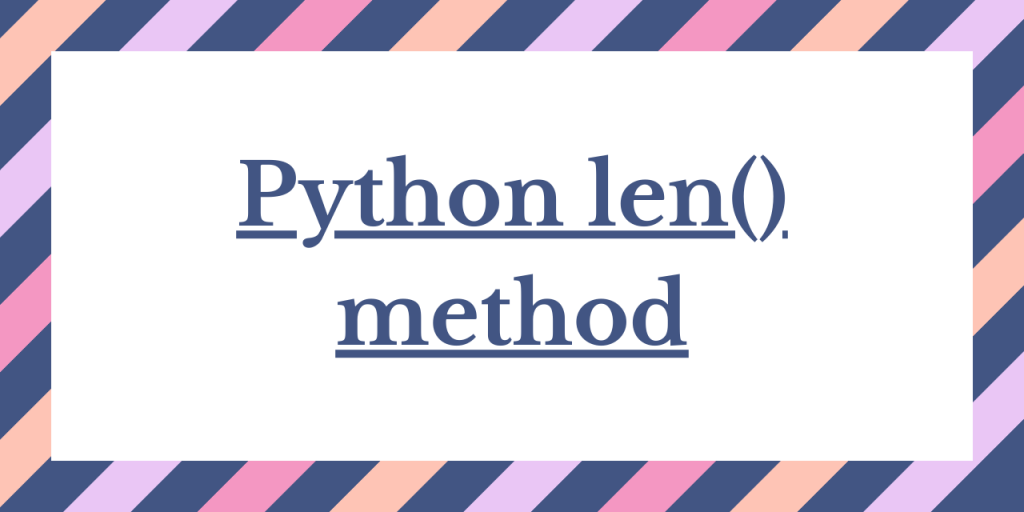# How to Find the Array Length in PythonPython len() method is used to find the length of an array. As we all know, python does not support or provide us with the array data structure in a direct way. Instead, python serves with three different variations of using an array data structure.

In this tutorial, we will learn about the fundamentals of the different array variants that can use to create an array in python and then we will discuss the use of the len() method to obtain the length of an array in each variant.

## Finding the length of an Array using the len() Method

We have three methods to create an array in Python, which we will use to demonstrate the use of the len() method.

To demonstrate the len() method, we’ll use all of these types of arrays, but before that let’s take a look at the syntax of len() method.

### len() Method in Python

`Python len() method` enables us to find the total number of elements in an array. It returns the count of the elements in an array.

Syntax:

```len(array)
```

Here, the array can be any type of array for which we want to find the length.

### Finding the Length of a Python List using len() Method

The `Python len() method` can be used to fetch and display the number of elements contained in the list.

Example:

In the below example, we have created a list of heterogeneous elements. Further, we have used len() method to display the length of the list.

```lst = [1,2,3,4,'Python']
print("List elements: ",lst)
print("Length of the list:",len(lst))
```

Output:

```List elements:  [1, 2, 3, 4, 'Python']
Length of the list: 5
```

### Finding the Length of a Python Array using len() Method

`Python Array module` helps us create an array and manipulate the same using various functions of the module. The len() method can also be used to calculate the length of an array created using the Array module.

Example:

```import array as A
arr = A.array('i',[1,2,3,4,5])
print("Array elements: ",arr)
print("Length of array: ",len(arr))
```

Output:

```Array elements:  array('i', [1, 2, 3, 4, 5])
Length of array: 5
```

### Finding the Length of a Python NumPy Array using len() Method

As we all know, we can create an array using `NumPy module` and use it for any mathematical purpose. The len() method helps us find out the number of data values present in a NumPy array.

Example:

```import numpy as np
arr = np.arange(5)
len_arr = len(arr)
print("Array elements: ",arr)
print("Length of NumPy array: ",len_arr)
```

Output:

```Array elements:  [0 1 2 3 4]
Length of NumPy array: 5
```

## Conclusion

In this tutorial, we learned to use the len() method to find the length of different arrays in Python. We have given you many examples so that you can learn them well. Hope you find this tutorial useful.

## Reference

https://stackoverflow.com/questions/518021/is-arr-len-the-preferred-way-to-get-the-length-of-an-array-in-python